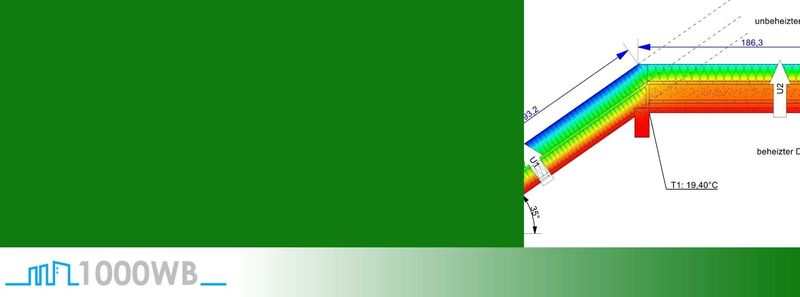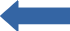# Reference lengths of the U-values – Example floor slab

Posted on: May 31, 2020 Posted by: Jörg Gerl Comments: 0Here we have a thermal bridge that has a precisely defined thermal bridge coefficient for a given geometry Ψ.

This applies to the undisturbed area (the area which has no influence on the heat flow from adjacent components):
– U1: U-value, which is used in the heat requirement calculation as the U-value of the outer wall (in this example: 0.1331 W/m²K)
– U2: U-value that is used in the heat requirement calculation as the U-value of the floor slab (in this example: 0.1392 W/m²K)

This geometry assumes that in the heat requirement calculation the undisturbed U-value, which is set for the floor slab, extends to the lower edge of the floor slab.

The dimensioning and reference lengths of the U-values correspond. It is not necessary to adjust the reference lengths of the U values.

However, if one now calculates the heat requirement according to the EnEV regulations, the reinforced concrete slab is not taken into account in this example for the reference length of the U-value of the floor slab.

The outer wall is only calculated up to the lower edge of the insulation layer. Its height is thus reduced by 26 cm. The total area of the outer wall is reduced accordingly for the heat requirement calculation.

Dimensioning and reference lengths of the U-values no longer match. They differ by the thickness of the base plate (26 cm). This results in an incorrect result in the heat requirement calculation. The reference lengths of the U-values must be adjusted.

The calculated energy requirement of the entire building changes. If the reference lengths of the U-values used in the thermal bridge calculation were not adjusted, an error would result.

By adjusting the reference lengths of the U-values (here -26 cm at U1), an incorrect result of the heat requirement calculation is avoided.
Dimensioning and reference length of the U-values correspond again.

Let us consider the matter arithmetically by inserting the values into the formula

ψ = L2D – (Σ Ui*lI*Fxi)

or here specifically:

ψ = L2D – (U1*l1*Fx1+ U2*l2*Fx2)

Case one:
In this example we have the following quantities:

L2D = 0.6553
U1 = 0.1331 W/m²K
l1 = 1.96 m
Fx1 = 1
U2 = 0.1827 W/m²K
l2 = 2.0493 m
Fx2 = 0.6

This results in Ψ = 0.1697 W/mK

Case two:
If we now change the reference lengths of the U-values by the thickness of the base plate, it looks like this:

L2D = 0.6553
U1 = 0.1331 W/m²K
l1 = 1.96 m – 0.26 m = 1.70 m
Fx1 = 1
U2 = 0.1827 W/m²K
l2 = 2.0493 m
Fx2 = 0.6

This results in Ψ = – 0.2044 W/mK

This value is significantly worse than the unchanged Ψ value and thus takes into account the fact that a smaller wall surface compared to the original case causes less energy loss.